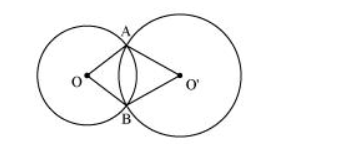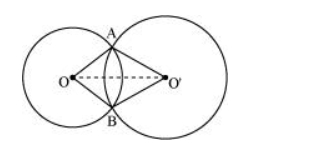# Prove that line of centres of two intersecting circles subtends

Question. Prove that line of centres of two intersecting circles subtends equal angles at the two points of intersection.

Solution:Let two circles having their centres as $O$ and $O^{\prime}$ intersect each other at point $A$ and $B$ respectively. Let us join $O O^{\prime}$.In $\triangle \mathrm{AO} \mathrm{O}^{\prime}$ and $\mathrm{BO} \mathrm{O}^{\prime}$,

OA $=$ OB (Radius of circle 1)

$\mathrm{O}^{\prime} \mathrm{A}=\mathrm{O}^{\prime} \mathrm{B}$ (Radius of circle 2)

$\mathrm{O} \mathrm{O}^{\prime}=\mathrm{O} \mathrm{O}^{\prime}$ (Common)

$\triangle \mathrm{AOO}^{\prime} \cong \triangle \mathrm{BOO}^{\prime}($ By SSS congruence rule $)$

$\angle O A O^{\prime}=\angle O B O^{\prime}($ By $C P C T)$

Therefore, line of centres of two intersecting circles subtends equal angles at the two points of intersection.